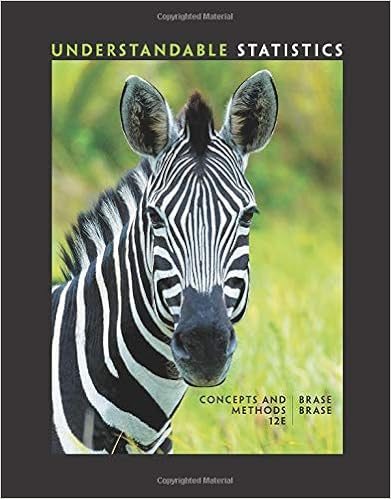# All of the following characteristics are common to both...

• Homework Help
• 10
• 100% (9) 9 out of 9 people found this document helpful

This preview shows page 1 - 3 out of 10 pages.

##### We have textbook solutions for you!
The document you are viewing contains questions related to this textbook.The document you are viewing contains questions related to this textbook.
Chapter 7 / Exercise 26
Understandable Statistics: Concepts and Methods
BraseExpert Verified
Principles of Microeconomics Spring 2018 Homework 9 Name Pratik Kacha MULTIPLE CHOICE. Choose the one alternative that best completes the statement or answers the question. 1) All of the following characteristics are common to both monopolistic competition and perfect competition except 1) D A) the market demand curves are downward - sloping. B)firms act to maximize profit. C) entry barriers into the industries are low. D) firms take market prices as given.
2) Which of the following is true for a firm with a downward - sloping demand curve for its product? 2) B
3) When a monopolistically competitive firm cuts its price to increase its sales, it experiences a loss in revenue due to the
1
##### We have textbook solutions for you!
The document you are viewing contains questions related to this textbook.The document you are viewing contains questions related to this textbook.
Chapter 7 / Exercise 26
Understandable Statistics: Concepts and Methods
BraseExpert Verified
Figure 13 - 2 4) Refer to Figure 13 - 2. The marginal revenue from selling the additional unit Q instead of Q a equals 4) C b + H) . H) .
5) For the monopolistically competitive firm 5) B A) P = AR > B) P > MR = C) Price ( P ) = Marginal Revenue ( MR ) = Average Revenue ( D) P = MR > MR . AR . AR ). AR .
Figure 13 - 3 6) Refer to Figure 13 - 3. What is the marginal revenue of the sixth unit of output?
•••